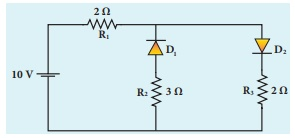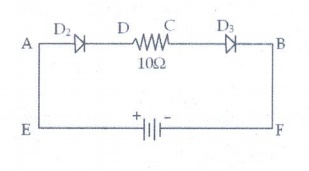Home | | Physics 12th Std | Exercise Numerical Problems

# Exercise Numerical Problems

Physics : Semiconductor Electronics: Book Back, Exercise, Example Numerical Question with Answers, Solution: Exercise Numerical Problems with Answers, Solution

Numerical Problems

1. The given circuit has two ideal diodes connected as shown in figure below. Calculate the current flowing through the resistance R1 [Ans: 2.5 A]Solution:

Barrier potential for ideal diode is zero. The diode D1 is reverse biased, so it will block the current and diode D2 is forward biased, so it will pass the current.

The given circuit becomesEffective resistance Reff = R1 + R3 = 4╬®

Current through R1 = V/Reff = 10/4 = 2.5A

2. Four silicon diodes and a 10 ╬® resistor are connected as shown in figure below. Each diode has a resistance of 1╬®. Find the current flows through the 18╬® resistor. [Ans: 0.13 A]Solution:

In the given circuit D2 & D3 are in forward bias so they conduct current while D1 &D4 are in reverse bias so they do not conduct current. So the equivalents circuit will bethe effective resistance is Reff

= 1╬® + 10╬® + 1╬® = 12╬®

Here silicon diodes are used

Ōł┤ Barrier potential for Si is 0.7 V

Net potential (Vnet) = 3 ŌĆō 0.7 - 0.7

Vnet = 1.6 V

Current (I) = Vnet / R eff = 1.6 / 12 = 0.133A

3. Assuming VCEsat = 0.2 V and ╬▓ = 50, find the minimum base current (IB) required to drive the transistor given in the figure to saturation. [Ans: 56 ┬ĄA]Solution;

VCE = 0.2V

Rc = 1k╬®

╬▓ =50

Vcc = 3V

I B = ?Ic = [ VCC ŌĆō VCE ] / RC

= (3-0.2) / 103

= 2.8 ├Ś 10-3 A

= 2.8 mA

╬▓= IC/IB

Ōł┤ IB = IC / ╬▓ = 2.8 ├Ś 10-3 / 50 = 0.056├Ś 10-3

= 56 ├Ś 10-6 A

IB=56╬╝A

4. A transistor having ╬▒ =0.99 and VBE = 0.7V, is given in the circuit. Find the value of the collector current.

[Ans: 5.33 mA]Given data:

╬▒ =0.99

VBE = 0.7V

IC=?

Solution:Tranistor is in saturation region.

Ōł┤ Ic = Ic(sat)

IC and IB are independent

VCE(sat) = 0.2 V, VBE(sat) = 0.8 V for silicon transistor (ie, standard value)

Apply KVR across B-E loop:

V1k + V10k + VBE sat + V1k = VCC

Ōł┤ 1(IC + IB) + 10 IB + 0.8 + 1 (IC + IB) = 12

2 IC + 12 IB = 11.2         ŌĆ”ŌĆ”ŌĆ”ŌĆ”ŌĆ”(1)

Apply KVR across C-E loop:

V1k + V1k + VCE sat + V1k = VCC

l(IC + IB) + 1 IC + 0.2 + I(IC + IB) = 12

3IC + 2IB = 11.8    ŌĆ”ŌĆ”ŌĆ”ŌĆ”ŌĆ”ŌĆ”.(2)

Solve the equation (1) and (2)

IB = 0.3125 mA

IC = 3.725 mA Ōēł 3.73 mA

5. In the circuit shown in the figure, the BJT has a current gain (╬▓) of 50. For an emitter ŌĆō base voltage VEB = 600 mV, calculate the emitter ŌĆō collector voltage VEC (in volts). [Ans: 2 V]Given data:

╬▓ = 50

VE=3V

VEB=60 mV

RB=60K╬®

RE=500╬®

Solution:

VB=VEŌłÆVEB

VB = 3ŌłÆ0.6 = 2.4V

IB = VB/RB = 2.4 / 60├Ś103 = 40╬╝A

IC = ╬▓IB = 50├Ś40╬╝A = 2├Ś10-3 A = 2mA

VC = RCIC = 500├Ś2├Ś10-3 = 1V

VEC = VE-VC = 3-1 = 2V

VEC = 2V

6. Determine the current flowing through 3╬® and 4╬® resistors of the circuit given below. Assume that diodes D1 and D2 are ideal diodes.Solution:

The diode D2 is in reverse biased. So does not conduct current.

Ōł┤ current through 3╬® is = 0

The diode D1 is in forward biased, and it is an ideal diode. So the given circuit becomes asThe current through 4╬® is

Ōł┤ I = V/R = 12/6 =2A

7. Prove the following Boolean expressions using the laws and theorems of Boolean algebra.

i) (A + B) (A +) = A

ii) A  (+B) = AB

iii) (A + B) (A + C) = A + BC

Solution:8. Verify the given Boolean equation A +  ßŠ╣B = A + B using truth table.

Solution:Hence, verified

9. In the given figure of a voltage regulator, a Zener diode of breakdown voltage 15V is employed. Determine the current through the load resistance, the total current and the current through the diode. Use diode approximation.Solution:

Voltage across RL(VO) = Vz = 15V

Voltage across RS(VRS) = 25 -15 = 10V

current through RL is

IL = V0/RL = 15 / 3├Ś103 = 5├Ś10-3 A

IL = 5 mA

Current through RS is

I = VRS/RS = 10/500 = 20├Ś10-3A

I = 20mA

Current through Zener diode is

IZ=I-IL

= (20-5) ├Ś 10-3

Iz = 15mA

10. Write down Boolean equation for the output Y of the given circuit and give its truth table.Solution:Tags : with Answers, Solution | Semiconductor Electronics | Physics , 12th Physics : UNIT 10a : Semiconductor Electronics
Study Material, Lecturing Notes, Assignment, Reference, Wiki description explanation, brief detail
12th Physics : UNIT 10a : Semiconductor Electronics : Exercise Numerical Problems | with Answers, Solution | Semiconductor Electronics | Physics